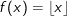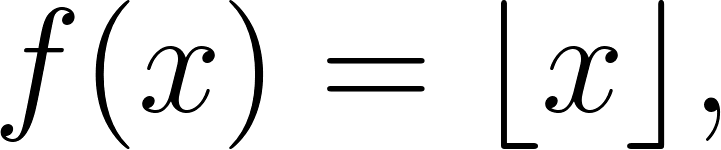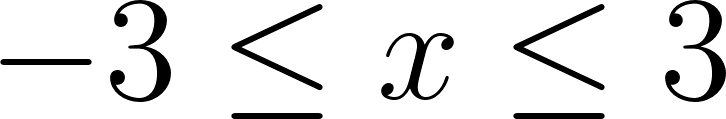×
Get Full Access to Calculus: Early Transcendentals - 1 Edition - Chapter 1.2 - Problem 48e
Get Full Access to Calculus: Early Transcendentals - 1 Edition - Chapter 1.2 - Problem 48e

×

# Floor function. The floor function, or greatest integerISBN: 9780321570567 2

## Solution for problem 48E Chapter 1.2

Calculus: Early Transcendentals | 1st Edition

• Textbook Solutions
• 2901 Step-by-step solutions solved by professors and subject experts
• Get 24/7 help from StudySoup virtual teaching assistantsCalculus: Early Transcendentals | 1st Edition

4 5 1 324 Reviews
30
5
Problem 48E

Floor function: The floor function, or greatest integer function,gives the greatest integer less than or equal to x. Graph the floor function for3 ≤ x ≤ 3.

Step-by-Step Solution:

Step 1 of 2

Given,

The Floor function,gives the greatest integer less than or equal to.

We need to find the Graph the floor function for.

Step 2 of 2

##### ISBN: 9780321570567

This textbook survival guide was created for the textbook: Calculus: Early Transcendentals, edition: 1. The answer to “?Floor function: The floor function, or greatest integer function, gives the greatest integer less than or equal to x. Graph the floor function for 3 ? x ? 3.” is broken down into a number of easy to follow steps, and 29 words. This full solution covers the following key subjects: floor, function, Integer, greatest, gives. This expansive textbook survival guide covers 85 chapters, and 5218 solutions. Calculus: Early Transcendentals was written by and is associated to the ISBN: 9780321570567. Since the solution to 48E from 1.2 chapter was answered, more than 386 students have viewed the full step-by-step answer. The full step-by-step solution to problem: 48E from chapter: 1.2 was answered by , our top Calculus solution expert on 03/03/17, 03:45PM.

Unlock Textbook Solution